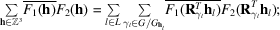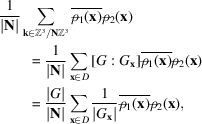International
Tables for
Crystallography
Volume B
Reciprocal space
Edited by U. Shmueli

International Tables for Crystallography (2010). Vol. B, ch. 1.3, p. 74   | 1 | 2 |

## Section 1.3.4.2.2.8. Parseval's theorem with crystallographic symmetry

G. Bricognea

aGlobal Phasing Ltd, Sheraton House, Suites 14–16, Castle Park, Cambridge CB3 0AX, England, and LURE, Bâtiment 209D, Université Paris-Sud, 91405 Orsay, France

#### 1.3.4.2.2.8. Parseval's theorem with crystallographic symmetry

| top | pdf |

The general statement of Parseval's theorem given in Section 1.3.4.2.1.5may be rewritten in terms of symmetry-unique structure factors and electron densities by means of orbit decomposition.

In reciprocal space,for each l, the summands corresponding to the variousare equal, so that the left-hand side is equal toIn real space, the triple integral may be rewritten as(where D is the asymmetric unit) ifandare smooth densities, since the set of special positions has measure zero. If, however, the integral is approximated as a sum over a G-invariant grid defined by decimation matrix N, special positions on this grid must be taken into account:where the discrete asymmetric unit D contains exactly one point in each orbit of G in.